6265.0 - Underemployed Workers, Australia, Sep 2008
ARCHIVED ISSUE Released at 11:30 AM (CANBERRA TIME) 24/02/2009
Page tools:Print All

TECHNICAL NOTE DATA QUALITY

INTRODUCTION

1 Estimates in this publication are based on information obtained from occupants of a sample of dwellings, and are subject to sampling variability. That is, they may differ from those estimates that would have been produced if all dwellings had been included in the survey. One measure of the likely difference is given by the standard error (SE), which indicates the extent to which an estimate might have varied by chance because only a sample of dwellings was included. There are about two chances in three (67%) that a sample estimate will differ by less than one SE from the number that would have been obtained if all dwellings had been included, and about 19 chances in 20 (95%) that the difference will be less than two SEs. Another measure of the likely difference is the relative standard error (RSE), which is obtained by expressing the SE as a percentage of the estimate.

2 Due to space limitations, it is impractical to print the SE of each estimate in the publication. Instead, a table of SEs is provided to determine the SE for an estimate from the size of that estimate (see table T1). The SE table is derived from a mathematical model, referred to as the 'SE model', which is created using data from a number of past Labour Force Surveys. It should be noted that the SE model only gives an approximate value for the SE for any particular estimate, since there is some minor variation between SEs for different estimates of the same size.

3 The LFS sample size in September 2008 was approximately one-third smaller than the sample size in September 2007. This is due to an 11% sample reduction that was implemented from November 2007 to June 2008 based on the 2006 sample design, and an additional 24% sample reduction implemented in July 2008. In combination, the two sample reductions are expected to increase the standard errors for estimates from the supplementary surveys by approximately 22% at the broad aggregate level, relative to the 2001 sample design (standard errors will vary at lower aggregate levels). Detailed information about the sample reduction is provided in Information Paper: Labour Force Survey Sample Design, Nov 2007 (Second edition) (cat. no. 6269.0).

CALCULATION OF STANDARD ERROR

4 An example of the calculation and the use of SEs in relation to estimates of persons is as follows. Table 5 shows the estimated number of female underemployed part-time workers was 389,800. Since this estimate is between 300,000 and 500,000, table T1 shows that the SE for Australia will lie between 7,600 and 9,550 and can be approximated by interpolation using the following general formula: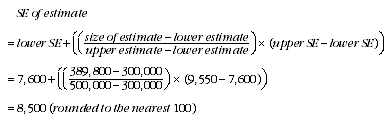5 Therefore, there are about two chances in three that the value that would have been produced if all dwellings had been included in the survey will fall within the range 381,300 to 398,300 and about 19 chances in 20 that the value will fall within the range 372,800 to 406,800. This example is illustrated in the following diagram.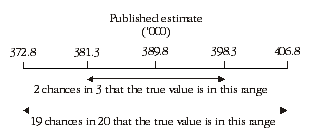6 In general, the size of the SE increases as the size of the estimate increases. Conversely, the RSE decreases as the size of the estimate increases. Very small estimates are thus subject to such high RSEs that their value for most practical purposes is unreliable. In the tables in this publication, only estimates with RSEs of 25% or less are considered reliable for most purposes. Estimates with RSEs greater than 25% but less than or equal to 50% are preceded by an asterisk (e.g.*3.2) to indicate they are subject to high SEs and should be used with caution. Estimates with RSEs of greater than 50%, preceded by a double asterisk (e.g.**0.3), are considered too unreliable for general use and should only be used to aggregate with other estimates to provide derived estimates with RSEs of less than 25%.

MEANS AND MEDIANS

7 The RSEs of estimates of mean duration of insufficient work, median duration of insufficient work and mean preferred number of extra hours are obtained by first finding the RSE of the estimate of the total number of persons contributing to the mean or median (see table T1) and then multiplying the resulting number by the following factors:
• mean duration of insufficient work: 1.6
• median duration of insufficient work: 2.5
• mean preferred number of extra hours: 0.7

8 The following is an example of the calculation of SEs where the use of a factor is required. Table 4 shows that the estimated number of male underemployed part-time workers was 213,900 with a median duration of insufficient work of 26 weeks. The SE of 213,900 can be calculated from table T1 (by interpolation) as 6,600. To convert this to an RSE we express the SE as a percentage of the estimate or 6,600/213,900 =3.1%.

9 The RSE of the estimate of median duration of insufficient work is calculated by multiplying this number (3.1%) by the appropriate factor shown in paragraph 7 (in this case 2.5): 3.1 x 2.5 = 7.8%. The SE of this estimate of median duration of insufficient work is therefore 7.8% of 26, i.e. about 2 (rounded to the nearest whole week). Therefore, there are two chances in three that the median duration of insufficient work for males that would have been obtained if all dwellings had been included in the survey would have been within the range 24-28 weeks, and about 19 chances in 20 that it would have been within the range 22-30 weeks.

PROPORTIONS AND PERCENTAGES

10 Proportions and percentages formed from the ratio of two estimates are also subject to sampling errors. The size of the error depends on the accuracy of both the numerator and the denominator. A formula to approximate the RSE of a proportion is given below. This formula is only valid when x is a subset of y.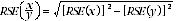11 Considering the example from paragraph 3, of the 389,800 female underemployed part-time workers, 131,100 or 33.6% had insufficient work for 52 weeks and over. The SE of 131,100 may be calculated by interpolation as 5,400. To convert this to an RSE we express the SE as a percentage of the estimate, or 5,400/131,100 = 4.1%. The SE for 389,800 was calculated previously as 8,500, which converted to an RSE is 8,500/389,800 = 2.2%. Applying the above formula, the RSE of the proportion is: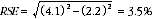12 Therefore, the SE for the proportion of females who have a current period of insufficient work of 52 weeks or more is 1.2 percentage points (=(33.6/100)x3.5). Therefore, there are about two chances in three that the proportion of females who have a current period of insufficient work of 52 weeks or more was between 32.4% and 34.8% and 19 chances in 20 that the proportion is within the range 31.2% to 36.0%.

DIFFERENCES

13 Published estimates may also be used to calculate the difference between two survey estimates (of numbers or percentages). Such an estimate is subject to sampling error. The sampling error of the difference between two estimates depends on their SEs and the relationship (correlation) between them. An approximate SE of the difference between two estimates (x-y) may be calculated by the following formula: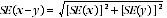14 While this formula will only be exact for differences between separate and uncorrelated characteristics or subpopulations, it is expected to provide a good approximation for all differences likely to be of interest in this publication.

STANDARD ERRORS

 T1 STANDARD ERRORS OF ESTIMATESAust.NSW Vic. Qld SA WA Tas. NT ACT SE RSESize of Estimate (persons) no. no. no. no. no. no. no. no. no. %100 340 330 250 200 250 130 90 120 120 120.0200 450 430 370 270 330 180 140 190 220 110.0300 540 510 450 320 390 220 170 240 300 100.0500 660 620 570 390 480 270 220 310 440 88.0700 760 710 670 450 550 310 260 350 550 78.61,000 880 810 780 520 630 360 300 380 700 70.01,500 1 030 950 930 600 730 410 350 420 890 59.32,000 1 150 1 060 1 040 670 820 450 390 440 1 050 52.52,500 1 250 1 150 1 150 750 900 500 400 450 1 200 48.03,000 1 350 1 250 1 200 800 950 500 450 500 1 300 43.33,500 1 450 1 300 1 300 800 1 000 550 450 500 1 400 40.04,000 1 500 1 400 1 350 850 1 050 550 500 550 1 500 37.55,000 1 650 1 500 1 450 950 1 150 600 550 600 1 700 34.07,000 1 850 1 700 1 650 1 050 1 300 700 650 650 1 950 27.910,000 2 150 1 950 1 850 1 200 1 500 800 800 800 2 300 23.015,000 2 500 2 250 2 050 1 350 1 700 950 1 150 950 2 650 17.720,000 2 750 2 500 2 250 1 500 1 900 1 150 1 450 1 100 2 950 14.830,000 3 200 2 900 2 600 1 800 2 150 1 450 2 000 1 450 3 350 11.240,000 3 550 3 200 2 850 2 050 2 400 1 700 2 550 1 700 3 650 9.150,000 3 850 3 500 3 150 2 300 2 650 1 950 3 050 1 900 3 900 7.8100,000 4 900 4 550 4 300 3 450 3 900 2 750 5 250 2 550 4 900 4.9150,000 5 750 5 550 5 300 4 400 5 150 3 300 7 250 2 900 5 700 3.8200,000 6 600 6 450 6 200 5 200 6 150 3 700 9 050 3 050 6 400 3.2300,000 8 300 8 300 7 850 6 400 7 750 4 200 12 350 3 200 7 600 2.5500,000 11 650 11 500 10 600 8 000 9 850 4 850 . . 3 200 9 550 1.91,000,000 17 300 17 500 15 150 10 200 12 600 5 550 . . . . 13 450 1.32,000,000 23 300 25 850 20 350 12 100 14 550 . . . . . . 19 550 1.05,000,000 29 700 41 350 27 450 13 650 15 200 . . . . . . 32 600 0.710,000,000 31 800 57 000 32 100 . . . . . . . . . . 43 500 0.415,000,000 . . . . . . . . . . . . . . . . 49 100 0.3. . not applicable